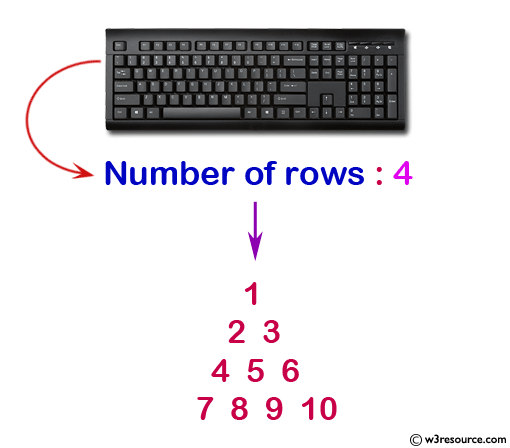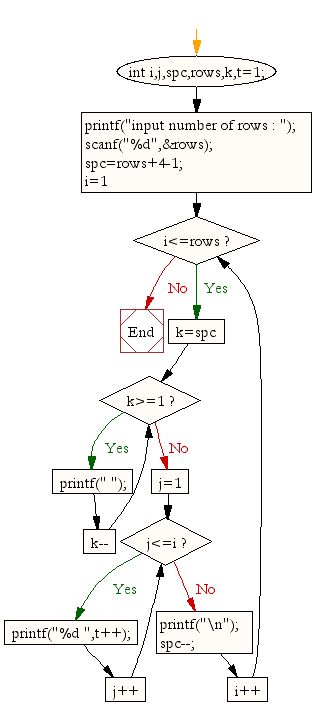﻿ C : Pyramid pattern with numbers increased by 1# C Exercises: Display the pattern like a pyramid with numbers increased by 1

## C For Loop: Exercise-13 with Solution

Write a program in C to make such a pattern like a pyramid with numbers increased by 1. The pattern is as follows:

```   1
2 3
4 5 6
7 8 9 10
```

Pictorial Presentation:Sample Solution:

C Code:

``````#include <stdio.h>
void main()
{
int i,j,spc,rows,k,t=1;
printf("Input number of rows : ");
scanf("%d",&rows);
spc=rows+4-1;
for(i=1;i<=rows;i++)
{
for(k=spc;k>=1;k--)
{
printf(" ");
}
for(j=1;j<=i;j++)
printf("%d ",t++);
printf("\n");
spc--;
}
}
```
```

Sample Output:

```Input number of rows : 4
1
2 3
4 5 6
7 8 9 10
```

Flowchart:C Programming Code Editor:

Improve this sample solution and post your code through Disqus.

What is the difficulty level of this exercise?

Test your Programming skills with w3resource's quiz.

﻿